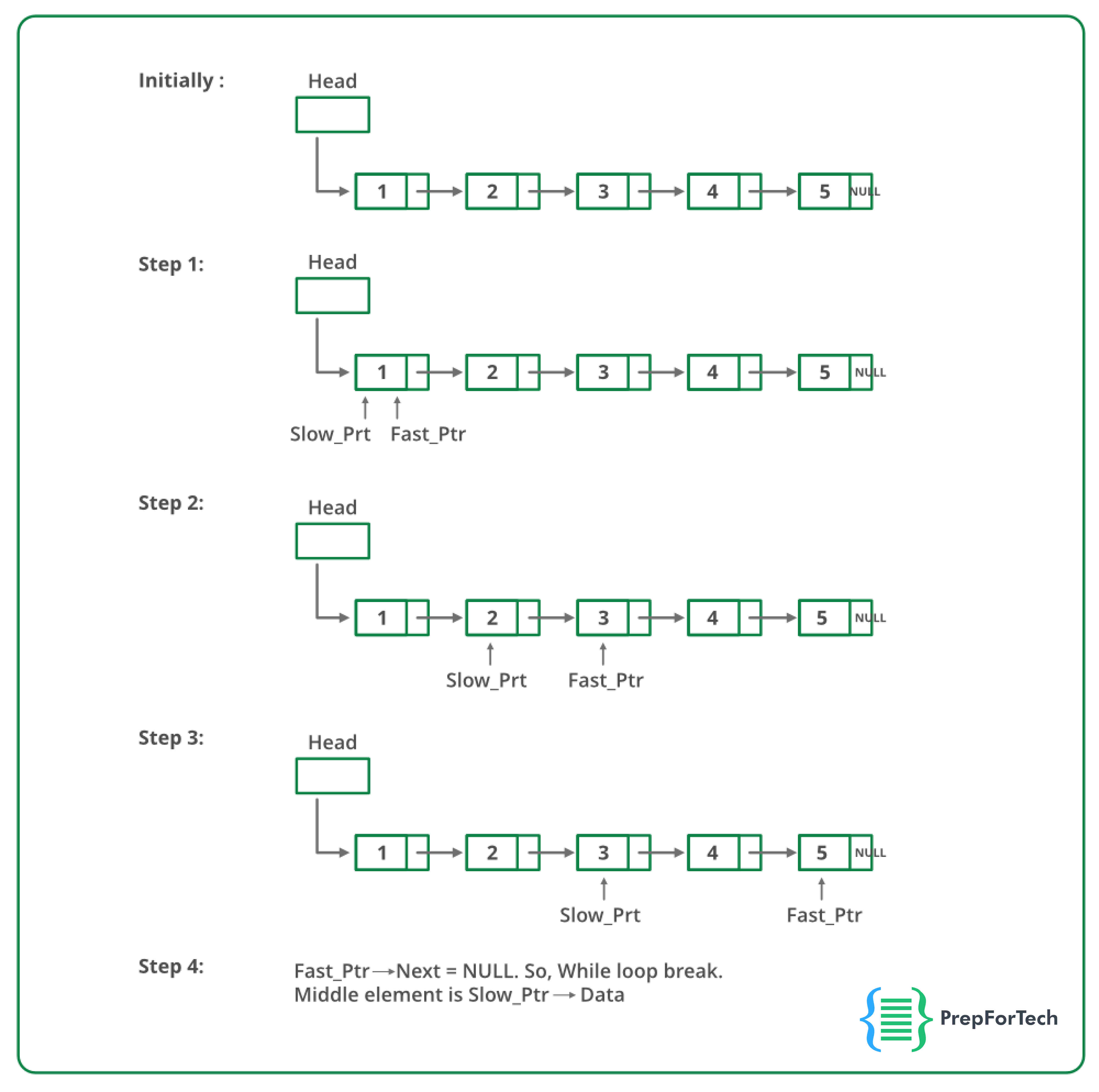# How to find middle of a linked list in one iteration ?

## How to find middle of a linked list in one iteration ?

There are multiple ways to find middle of a linked list. In this article, we discuss the two approaches to find the middle element of the linked list.

You can also see the below video, if you want to understand the approaches using a video solution.

### Approach 1

In this approach, we will find `n` which is the size of the linked list. Once we find `n`, we know the middle element will be located at position `n/2`. This approach requires two iterations :

1. First iteration for finding the value of `n`.
2. Second iteration for travelling to the middle of the linked list.

This approach’s time complexity is `O(n)` but the number of iterations is still 2.

### Approach 2 (Single Iteration)

This approach uses two pointer method in which we create two pointers. One fast pointer and one slow pointer.
In every iteration, the slow pointer moves one step at a time and the fast pointer moves two steps at a time. Once the fast pointer reaches the end, the slow pointer would be at the middle of the linked list.

#### Proof

Since, the slow pointer moves one step at a time and the fast pointer moves two steps at a time, after `x` iterations, the slow pointer would have moved to `x` position and the fast pointer would have moved to `2x` position.
Therefore, when the fast pointer reaches the end `2x` becomes equal to `n`.
`=> 2x = n`
`=> x = n/2`
Therefore, when the fast pointer reaches the end , the slow pointer is at position `x` which is `n/2`, which is the middle of the linked list.

### Algorithm

Step 1: Initialize two pointers one fast_pointer, and one slow_pointer to the start of the linked list.
Step 2: While fast_pointer does not reach the end do the following :
Step 3: Move slow_pointer by one step and fast_pointer by two steps

See the flowchart below for the steps:### Implementation

``````// C++ Program to detect loop in a linked list
#include <bits/stdc++.h>
using namespace std;

/* Link list node */
class Node {
public:
int data;
Node* next;
};

void push(Node** head_ref, int new_data)
{
/* allocate node */
Node* new_node = new Node();

/* put in the data */
new_node->data = new_data;

/* link the old list off the new node */
new_node->next = (*head_ref);

/* move the head to point to the new node */
(*head_ref) = new_node;
}

int findLoop(Node* list)
{
Node *slow_pointer = list, *fast_pointer = list;

while (slow_pointer && fast_pointer && fast_pointer->next) {
slow_pointer = slow_pointer->next;
fast_pointer = fast_pointer->next->next;
if (slow_pointer == fast_pointer) {
cout << "Found Loop";
return 1;
}
}
return 0;
}

int main()
{
/* Start with the empty list */
Node* head = NULL;

push(&head, 20);
push(&head, 4);
push(&head, 15);
push(&head, 10);

/* Create a loop for testing */
head->next->next->next->next = head;
detectloop(head);

return 0;
}``````
Scroll to Top

### Full Stack Integrated Bootcamp Free Trial

• Please enter a number from 7000000000 to 9999999999.